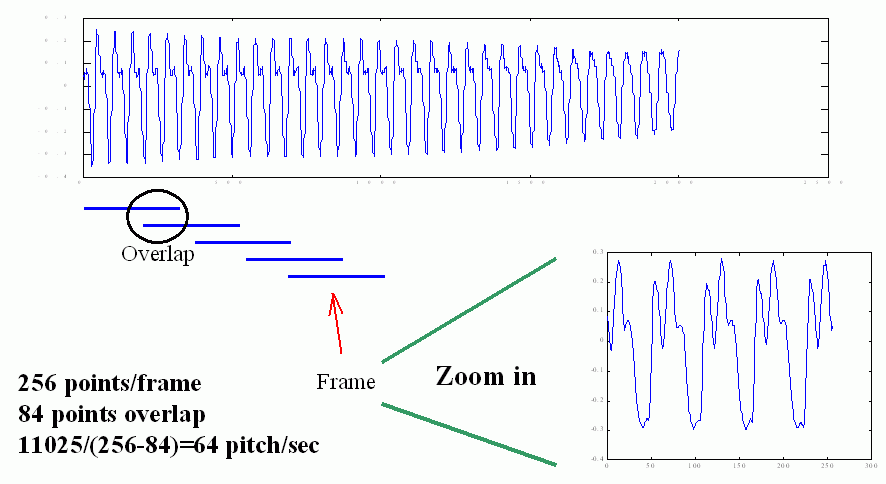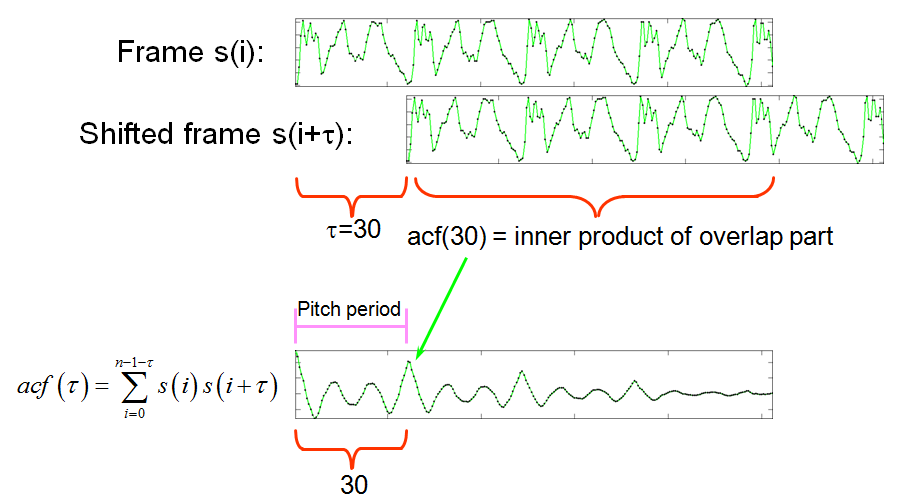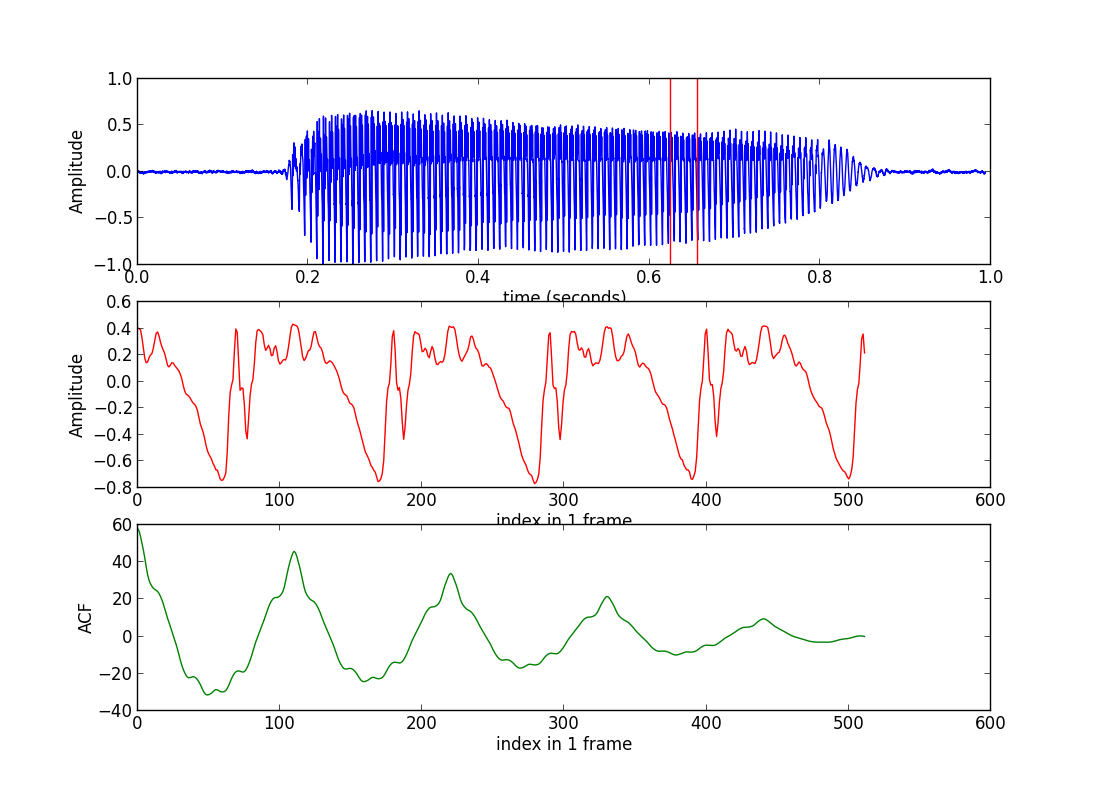## 1.概述

·旋律识别(Melody Recognition)：或称为“哼唱选歌”，也就是如何由使用者的哼唱，找出音乐资料库中间对应的歌。
·汉语声调识别(Tone Recognition)：辨识使用者讲一句话时，每一个字的声调（一声、二声、三声、四声等）。
·语音合成韵律分析(Prosody Analysis)中的音高分析：如何在合成语音时，使用最自然的音高曲线。
·语音评分中的音调评分(Intonation Assessment)：如何评估使用者说话的语音，其音高曲线是否标准。
·语音识别(Speech Recognition)：我们可以使用语句的音高来提高语音辨识的正确率。

## 2.音高追踪的基本流程

(1)将整段音讯讯号切成音框（Frames），相邻音框之间可以重叠。
(2)算出每个音框所对应的音高。
(3)排除不稳定的音高值。（可由音量来筛选，或由音高值的范围来过滤。）
(4)对整段音高进行平滑化，通常是使用「中位数滤波器」（Median Filters）。·音框长度至少必须包含2 个基本周期以上，才能显示语音的特性。已知人声的音高范围大约在50 Hz 至1000 Hz 之间，因此对于一个的取样频率，我们就可以计算出 音框长度的最小值。例如，若取样频率fs = 8000 Hz，那么当音高f = 50 Hz（例如男低音的歌声）时，每个基本周期的点数是fs/f = 8000/50 = 160，因此音框必须 至少是320 点；若音高是1000 Hz（例如女高音的歌声）时，每个基本周期的点数是8000/1000 = 8，因此音框必须至少是16 点。
·音框长度也不能太大，太长的音框无法抓到音讯的特性随时间而变化的细微现象，同时计算量也会变大。
·音框之间的重叠完全是看计算机的运算能力来决定，若重叠多，音框率就会变大，计算量就跟着变大。若重叠少（甚至可以不重叠或跳点），音框率就会变小， 计算量也跟着变小。

## 3.音高追踪算法

#### 3.1概述

·ACF: Autocorrelation function，自相关函数
·AMDF: Average magnitude difference function，平均强度差分函数
·SIFT: Simple inverse filter tracking

·Harmonic product spectrum method
·Cepstrum method

#### 3.2 ACF自相关函数

$acf(\tau) = \sum_{i=0}^{n-1-\tau}s(i)s(i+\tau)$$acf(\tau) = \sum{i=0}^{n-1-\tau} \frac{s(i)s(i+\tau)}{n-\tau}$.

$acf(\tau) = \sum{i=0}^{n/2}s(i)s(i+\tau)$.

#### 3.3 NSDF

ACF的范围是未知的，NSDF(normalized squared difference function)将ACF规整到[-1,1]之间，其定义的表达式如下：

$nsdf(\tau) = \frac{2\sum s(i)s(i+\tau)}{\sum s^{2}(i) + \sum s^{2}(i+\tau)}$.

#### 3.4 AMDF

AMDF (average magnitude difference function) 的定义如下：

$amdf(\tau) = \sum_{i=0}^{n-1-\tau}|s(i)-s(i+\tau)|$.

#### 3.5 Pitch Tracking

PS：另外，还有一些频域的音高追踪方法，将在后续文章中介绍。

## 4.参考资料

Audio Signal Processing and Recognition, Chap 7: http://neural.cs.nthu.edu.tw/jang/books/audiosignalprocessing/index.asp